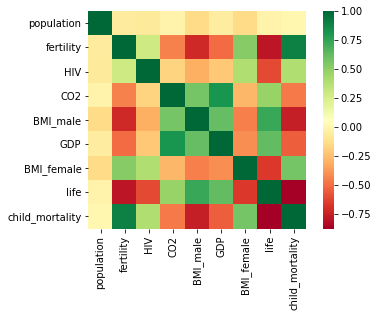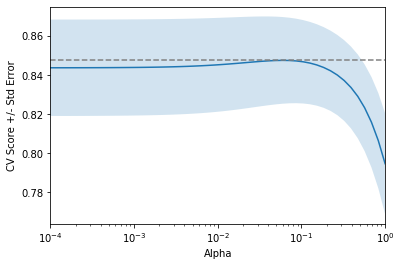import numpy as np
import pandas as pd
import matplotlib.pyplot as plt
import seaborn as sns


## Introduction to Regression

### Importing data for supervised learning

In this chapter, you will work with Gapminder data that we have consolidated into one CSV file available in the workspace as 'gapminder.csv'. Specifically, your goal will be to use this data to predict the life expectancy in a given country based on features such as the country's GDP, fertility rate, and population. As in Chapter 1, the dataset has been preprocessed.

Since the target variable here is quantitative, this is a regression problem. To begin, you will fit a linear regression with just one feature: 'fertility', which is the average number of children a woman in a given country gives birth to. In later exercises, you will use all the features to build regression models.

Before that, however, you need to import the data and get it into the form needed by scikit-learn. This involves creating feature and target variable arrays. Furthermore, since you are going to use only one feature to begin with, you need to do some reshaping using NumPy's .reshape() method. Don't worry too much about this reshaping right now, but it is something you will have to do occasionally when working with scikit-learn so it is useful to practice.

df = pd.read_csv('./dataset/gm_2008_region.csv')
df.drop(labels=['Region'], axis='columns', inplace=True)

# Create arrays for features and target variable
y = df['life'].values
X = df['fertility'].values

# Print the dimensions of X and y before reshaping
print("Dimensions of y before reshaping: {}".format(y.shape))
print("Dimensions of X before reshaping: {}".format(X.shape))

# Reshape X and y
y = y.reshape(-1, 1)
X = X.reshape(-1, 1)

# Print the dimensions of X and y after reshaping
print("Dimensions of y after reshaping: {}".format(y.shape))
print("Dimensions of X after reshaping: {}".format(X.shape))

Dimensions of y before reshaping: (139,)
Dimensions of X before reshaping: (139,)
Dimensions of y after reshaping: (139, 1)
Dimensions of X after reshaping: (139, 1)


### Exploring the Gapminder data

As always, it is important to explore your data before building models. On the right, we have constructed a heatmap showing the correlation between the different features of the Gapminder dataset. Cells that are in green show positive correlation, while cells that are in red show negative correlation. Take a moment to explore this: Which features are positively correlated with life, and which ones are negatively correlated? Does this match your intuition?

sns.heatmap(df.corr(), square=True, cmap='RdYlGn')

<matplotlib.axes._subplots.AxesSubplot at 0x286a8e37108>df.describe()

population fertility HIV CO2 BMI_male GDP BMI_female life child_mortality
count 1.390000e+02 139.000000 139.000000 139.000000 139.000000 139.000000 139.000000 139.000000 139.000000
mean 3.549977e+07 3.005108 1.915612 4.459874 24.623054 16638.784173 126.701914 69.602878 45.097122
std 1.095121e+08 1.615354 4.408974 6.268349 2.209368 19207.299083 4.471997 9.122189 45.724667
min 2.773150e+05 1.280000 0.060000 0.008618 20.397420 588.000000 117.375500 45.200000 2.700000
25% 3.752776e+06 1.810000 0.100000 0.496190 22.448135 2899.000000 123.232200 62.200000 8.100000
50% 9.705130e+06 2.410000 0.400000 2.223796 25.156990 9938.000000 126.519600 72.000000 24.000000
75% 2.791973e+07 4.095000 1.300000 6.589156 26.497575 23278.500000 130.275900 76.850000 74.200000
max 1.197070e+09 7.590000 25.900000 48.702062 28.456980 126076.000000 135.492000 82.600000 192.000000
df.info()

<class 'pandas.core.frame.DataFrame'>
RangeIndex: 139 entries, 0 to 138
Data columns (total 9 columns):
#   Column           Non-Null Count  Dtype
---  ------           --------------  -----
0   population       139 non-null    float64
1   fertility        139 non-null    float64
2   HIV              139 non-null    float64
3   CO2              139 non-null    float64
4   BMI_male         139 non-null    float64
5   GDP              139 non-null    float64
6   BMI_female       139 non-null    float64
7   life             139 non-null    float64
8   child_mortality  139 non-null    float64
dtypes: float64(9)
memory usage: 9.9 KB


## The basics of linear regression

• Regression mechanics
• $y = ax + b$
• $y$ = target
• $x$ = single feature
• $a, b$ = parameters of model
• Define an error functions for any given line
• Choose the line that minimizes the error function
• The loss function
• Ordinary least squares (OLD) : Minimize sum of squares of residuals
• Linear regression in higher dimensions $$y = a_1 x_1 + a_2 x_2 + b$$
• To fit a linear regression model here:
• Need to specify 3 variables
• In higher dimensions:
• Must specify coefficient for each feature and the variable b $y = a_1x_1 + a_2x_2 + a_3x_3 + \dots + a_nx_n + b$

### Fit & predict for regression

Now, you will fit a linear regression and predict life expectancy using just one feature. You saw Andy do this earlier using the 'RM' feature of the Boston housing dataset. In this exercise, you will use the 'fertility' feature of the Gapminder dataset. Since the goal is to predict life expectancy, the target variable here is 'life'.

X_fertility = df['fertility'].values.reshape(-1, 1)
y = df['life'].values.reshape(-1, 1)

sns.scatterplot(x='fertility', y='life', data=df)

<matplotlib.axes._subplots.AxesSubplot at 0x286ab6c7cc8>As you can see, there is a strongly negative correlation, so a linear regression should be able to capture this trend. Your job is to fit a linear regression and then predict the life expectancy, overlaying these predicted values on the plot to generate a regression line. You will also compute and print the $R^2$ score using sckit-learn's .score() method.

from sklearn.linear_model import LinearRegression

# Create the regressor: reg
reg = LinearRegression()

# Create th prediction space
prediction_space = np.linspace(min(X_fertility), max(X_fertility)).reshape(-1, 1)

# Fit the model to the data
reg.fit(X_fertility, y)

# compute predictions over the prediction space: y_pred
y_pred = reg.predict(prediction_space)

# Print $R^2$
print(reg.score(X_fertility, y))

# Plot regression line on scatter plot
sns.scatterplot(x='fertility', y='life', data=df)
plt.plot(prediction_space, y_pred, color='black', linewidth=3)

0.6192442167740037

[<matplotlib.lines.Line2D at 0x286ab838208>]### Train/test split for regression

Train and test sets are vital to ensure that your supervised learning model is able to generalize well to new data. This was true for classification models, and is equally true for linear regression models.

In this exercise, you will split the Gapminder dataset into training and testing sets, and then fit and predict a linear regression over all features. In addition to computing the $R^2$ score, you will also compute the Root Mean Squared Error (RMSE), which is another commonly used metric to evaluate regression models.

from sklearn.linear_model import LinearRegression
from sklearn.metrics import mean_squared_error
from sklearn.model_selection import train_test_split

# Create training and test sets
X_train, X_test, y_train, y_test = train_test_split(X, y, test_size=0.3, random_state=42)

# Create the regressor: reg_all
reg_all = LinearRegression()

# Fit the regressor to the training data
reg_all.fit(X_train, y_train)

# Predict on the test data: y_pred
y_pred = reg_all.predict(X_test)

# compute and print R^2 and RMSE
print("R^2: {}".format(reg_all.score(X_test, y_test)))
rmse = np.sqrt(mean_squared_error(y_test, y_pred))
print("Root Mean Squared Error: {}".format(rmse))

R^2: 0.7298987360907494
Root Mean Squared Error: 4.194027914110243


## Cross-validation

• Cross-validation motivation
• Model performance is dependent on way the data is split
• Not representative of the model's ability to generalize
• Solution : Cross-validation!
• k-fold Cross-validation

### 5-fold cross-validation

Cross-validation is a vital step in evaluating a model. It maximizes the amount of data that is used to train the model, as during the course of training, the model is not only trained, but also tested on all of the available data.

In this exercise, you will practice 5-fold cross validation on the Gapminder data. By default, scikit-learn's cross_val_score() function uses $R^2$ as the metric of choice for regression. Since you are performing 5-fold cross-validation, the function will return 5 scores. Your job is to compute these 5 scores and then take their average.

from sklearn.model_selection import cross_val_score

# Create a linear regression object: reg
reg = LinearRegression()

# Compute 5-fold cross-validation scores: cv_scores
cv_scores = cross_val_score(reg, X, y, cv=5)

# Print the 5-fold cross-validation scores
print(cv_scores)

print("Average 5-Fold CV Score: {}".format(np.mean(cv_scores)))

[0.71001079 0.75007717 0.55271526 0.547501   0.52410561]
Average 5-Fold CV Score: 0.6168819644425119


### K-Fold CV comparison

Cross validation is essential but do not forget that the more folds you use, the more computationally expensive cross-validation becomes. In this exercise, you will explore this for yourself. Your job is to perform 3-fold cross-validation and then 10-fold cross-validation on the Gapminder dataset.

In the IPython Shell, you can use %timeit to see how long each 3-fold CV takes compared to 10-fold CV by executing the following cv=3 and cv=10:

%timeit cross_val_score(reg, X, y, cv = ____)

reg = LinearRegression()

# Perform 3-fold CV
%timeit cross_val_score(reg, X, y, cv=3)
cvscores_3 = cross_val_score(reg, X, y, cv=3)
print(np.mean(cvscores_3))

# Perform 10-fold CV
%timeit cross_val_score(reg, X, y, cv=10)
cvscores_10 = cross_val_score(reg, X, y, cv=10)
print(np.mean(cvscores_10))

1.98 ms ± 36.1 µs per loop (mean ± std. dev. of 7 runs, 100 loops each)
0.6294715754653507
6.27 ms ± 230 µs per loop (mean ± std. dev. of 7 runs, 100 loops each)
0.5883937741571185


## Regularized regression

• Why regularize?
• Recall: Linear regression minimizes a loss function
• It chooses a coefficient for each feature variable
• Large coefficient can lead to overfitting
• Penalizing large coefficients : Regularization
• Ridge regression
• Loss function = $\text{OLS loss function} + \alpha \sum^{n}_{i=1}a_i^2$
• Alpha : Parameter we need to choose (Hyperparameter or $\lambda$)
• Picking alpha is similar to picking k in k-NN
• Alpha controls model complexity
• Alpha = 0: get back OLS (Can lead to overfitting)
• Very high alpha: Can lead to underfitting
• Lasso regression
• Loss function = $\text{OLS loss function} + \alpha \sum^{n}_{i=1}|a_i|$
• Can be used to select import features of a dataset
• Shrinks the coefficients of less important features to exactly 0

### Regularization I: Lasso

In the video, you saw how Lasso selected out the 'RM' feature as being the most important for predicting Boston house prices, while shrinking the coefficients of certain other features to 0. Its ability to perform feature selection in this way becomes even more useful when you are dealing with data involving thousands of features.

In this exercise, you will fit a lasso regression to the Gapminder data you have been working with and plot the coefficients. Just as with the Boston data, you will find that the coefficients of some features are shrunk to 0, with only the most important ones remaining.

X = df.drop('life', axis='columns').values
y = df['life'].values

from sklearn.linear_model import Lasso

# Instantiate a lasso regressor: lasso
lasso = Lasso(alpha=0.4, normalize=True)

# Fit the regressor to the data
lasso.fit(X, y)

# Compute and print the coefficients
lasso_coef = lasso.coef_
print(lasso_coef)

# Plot the coefficients
df_columns = df.columns[:-1]
plt.plot(range(len(df_columns)), lasso_coef)
plt.xticks(range(len(df_columns)), df_columns.values, rotation=60)
plt.margins(0.02)

[-0.         -0.         -0.          0.          0.          0.
-0.         -0.07087587]### Regularization II: Ridge

Lasso is great for feature selection, but when building regression models, Ridge regression should be your first choice.

Recall that lasso performs regularization by adding to the loss function a penalty term of the absolute value of each coefficient multiplied by some alpha. This is also known as L1 regularization because the regularization term is the L1 norm of the coefficients. This is not the only way to regularize, however.

If instead you took the sum of the squared values of the coefficients multiplied by some alpha - like in Ridge regression - you would be computing the L2 norm. In this exercise, you will practice fitting ridge regression models over a range of different alphas, and plot cross-validated R2 scores for each.

def display_plot(cv_scores, cv_scores_std):
fig = plt.figure()
ax.plot(alpha_space, cv_scores)

std_error = cv_scores_std / np.sqrt(10)

ax.fill_between(alpha_space, cv_scores + std_error, cv_scores - std_error, alpha=0.2)
ax.set_ylabel('CV Score +/- Std Error')
ax.set_xlabel('Alpha')
ax.axhline(np.max(cv_scores), linestyle='--', color='.5')
ax.set_xlim([alpha_space, alpha_space[-1]])
ax.set_xscale('log')

from sklearn.linear_model import Ridge

# Setup the array of alphas and lists to store scores
alpha_space = np.logspace(-4, 0, 50)
ridge_scores = []
ridge_scores_std = []

# Create a ridge regressor: ridge
ridge = Ridge(normalize=True)

# Compute scores over range of alphas
for alpha in alpha_space:

# Specify the alpha value to use: ridge.alhpa
ridge.alpha = alpha

# Perform 10-fold CV: ridge_cv_scores
ridge_cv_scores = cross_val_score(ridge, X, y, cv=10)

# Append the mean of ridge_cv_scores to ridge_scores
ridge_scores.append(np.mean(ridge_cv_scores))

# Append the std of ridge_cv_scores to ridge_scores_std
ridge_scores_std.append(np.std(ridge_cv_scores))

# Display the plot
display_plot(ridge_scores, ridge_scores_std)